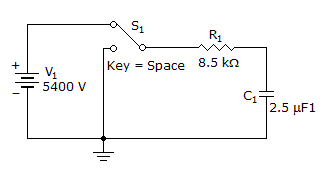# Electronics - Capacitors

### Exercise :: Capacitors - General Questions

41.

What is the voltage across a capacitor after being charged from a 100 V source for a period of one time constant?

 A. 37.8 V B. 38 V C. 63 V D. 101 V

Explanation:

No answer description available for this question. Let us discuss.

42.

What is the dc charging/discharging time constant for the circuit?A. 294 ps B. 13.5 ms C. 21.25 ms D. 2.16 Gs

Explanation:

No answer description available for this question. Let us discuss.

43.

Power stored from the source and then returned to the source is called:

 A. apparent power B. impedance power C. reactive power D. true power

Explanation:

No answer description available for this question. Let us discuss.

44.

Capacitor value markings are usually:

 A. alphanumeric B. color dabs C. standard D. white print

Explanation:

No answer description available for this question. Let us discuss.

45.

A source voltage across a capacitor will:

 A. lead the current by 90 degrees B. lead the current by 180 degrees C. lag the current by 90 degrees D. lag the current by 180 degrees